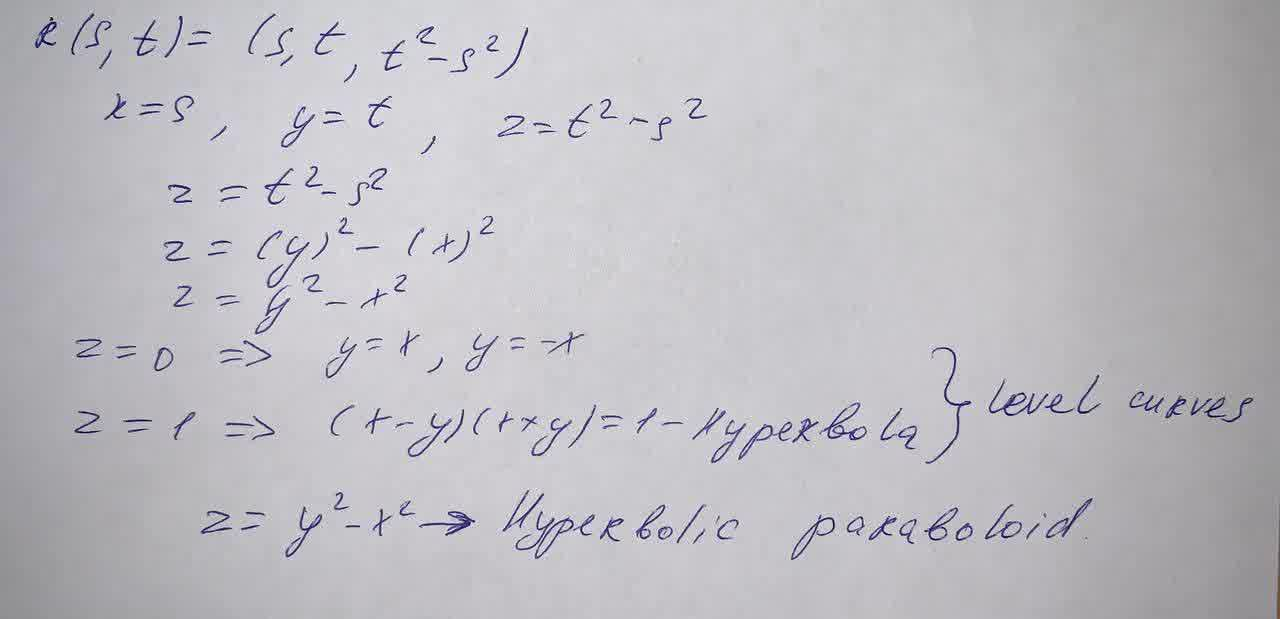Question# Identify the surface with the given vector equation.r(s,t)=(s,t,t^2-s^2). eliptic cylinder, circular paraboloid ,hyperbolic paraboloid

Vectors and spaces
ANSWEREDIdentify the surface with the given vector equation. r(s,t)=$$\displaystyle{\left({s},{t},{t}^{{2}}-{s}^{{2}}\right)}$$
eliptic cylinder
circular paraboloid
hyperbolic paraboloid
plane
circular cylinder